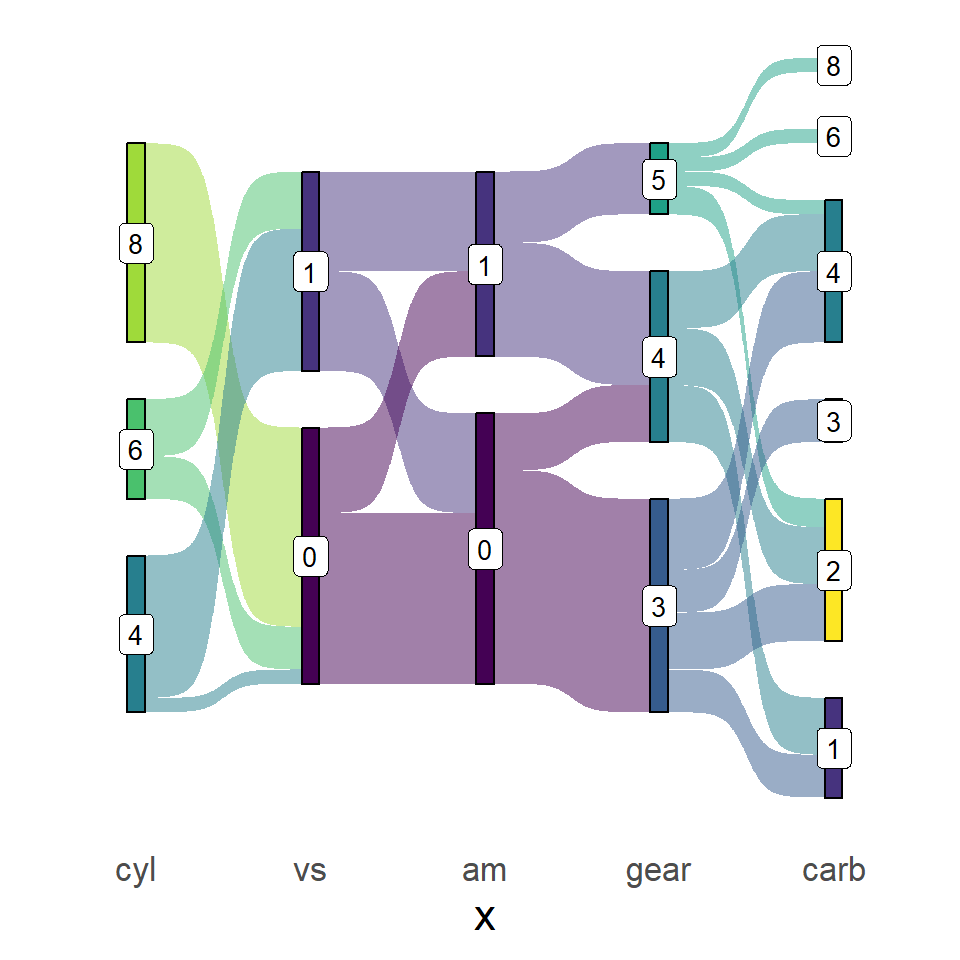# Sankey diagrams in ggplot2 with ggsankey

David Sjoberg

## Sample data set

In order to create a Sankey diagram in ggplot2 you will need to install the `ggsankey` library and transform your dataset using the `make_long` function from the package. The columns of the data must represent `x` (the current stage), `next_x` (the following stage), `node` (the current node) and `next_node` (the following node). Note that the last stage should point to an `NA`.

``````# install.packages("remotes")
# remotes::install_github("davidsjoberg/ggsankey")
library(ggsankey)

df <- mtcars %>%
make_long(cyl, vs, am, gear, carb)``````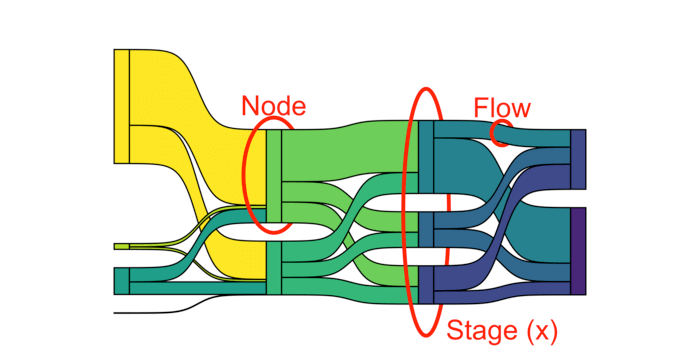## Sankey plot with `ggsankey`

The `ggsankey` package contains a geom named `geom_sankey` to create the Sankey diagrams in ggplot2. Note that you will need to pass the variables to `aes`, specifying a factor as the fill color. The function also contains a theme named `theme_sankey`.

``````# install.packages("remotes")
# remotes::install_github("davidsjoberg/ggsankey")
library(ggsankey)
# install.packages("ggplot2")
library(ggplot2)
# install.packages("dplyr")
library(dplyr) # Also needed

ggplot(df, aes(x = x,
next_x = next_x,
node = node,
next_node = next_node,
fill = factor(node))) +
geom_sankey() +
theme_sankey(base_size = 16)``````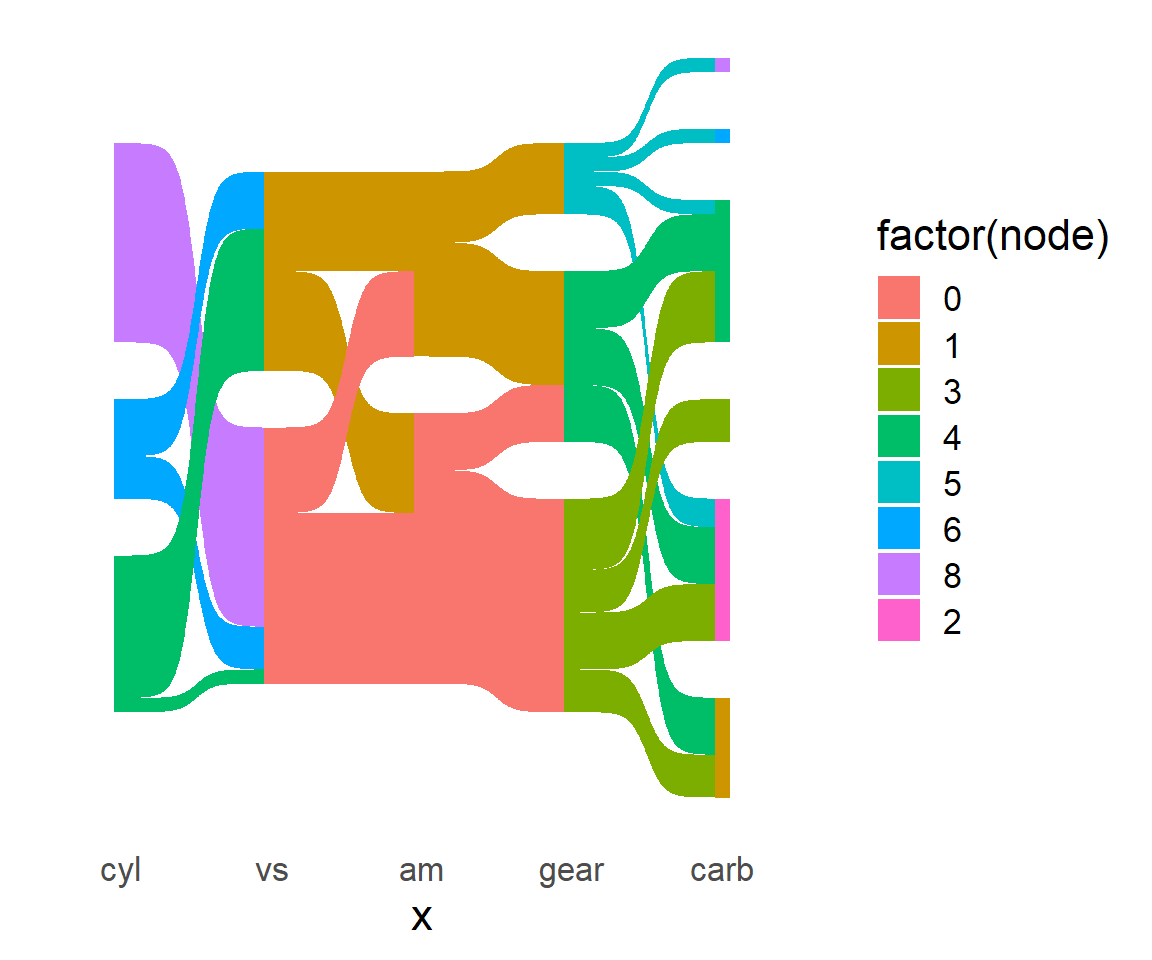You can also add labels to the Sankey diagram using the `geom_sankey_label` function of the package. Recall to specify the `label` inside `aes` as the variable you want to show.

``````# install.packages("remotes")
# remotes::install_github("davidsjoberg/ggsankey")
library(ggsankey)
# install.packages("ggplot2")
library(ggplot2)
# install.packages("dplyr")
library(dplyr) # Also needed

ggplot(df, aes(x = x,
next_x = next_x,
node = node,
next_node = next_node,
fill = factor(node),
label = node)) +
geom_sankey() +
geom_sankey_label() +
theme_sankey(base_size = 16)``````## Color customization

There are several arguments that can be customized to change the appearance of the Sankey diagram in R. The following images were created by the author of the package to serve as reference: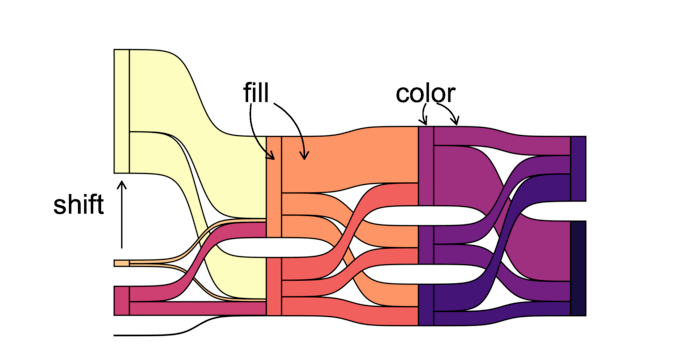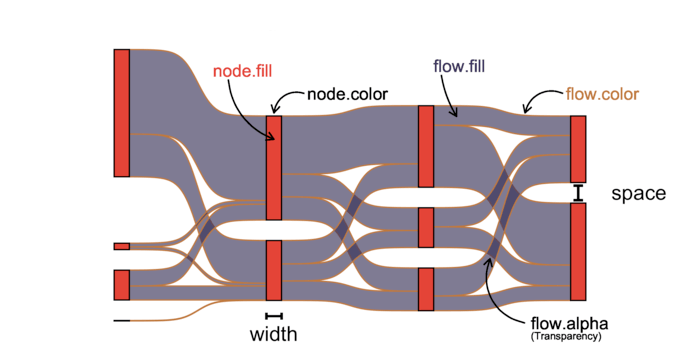Color and fill of the Sankey plot

As an example, modifying some arguments of the `geom_sankey` function and changing the fill color palette we can create something like the following: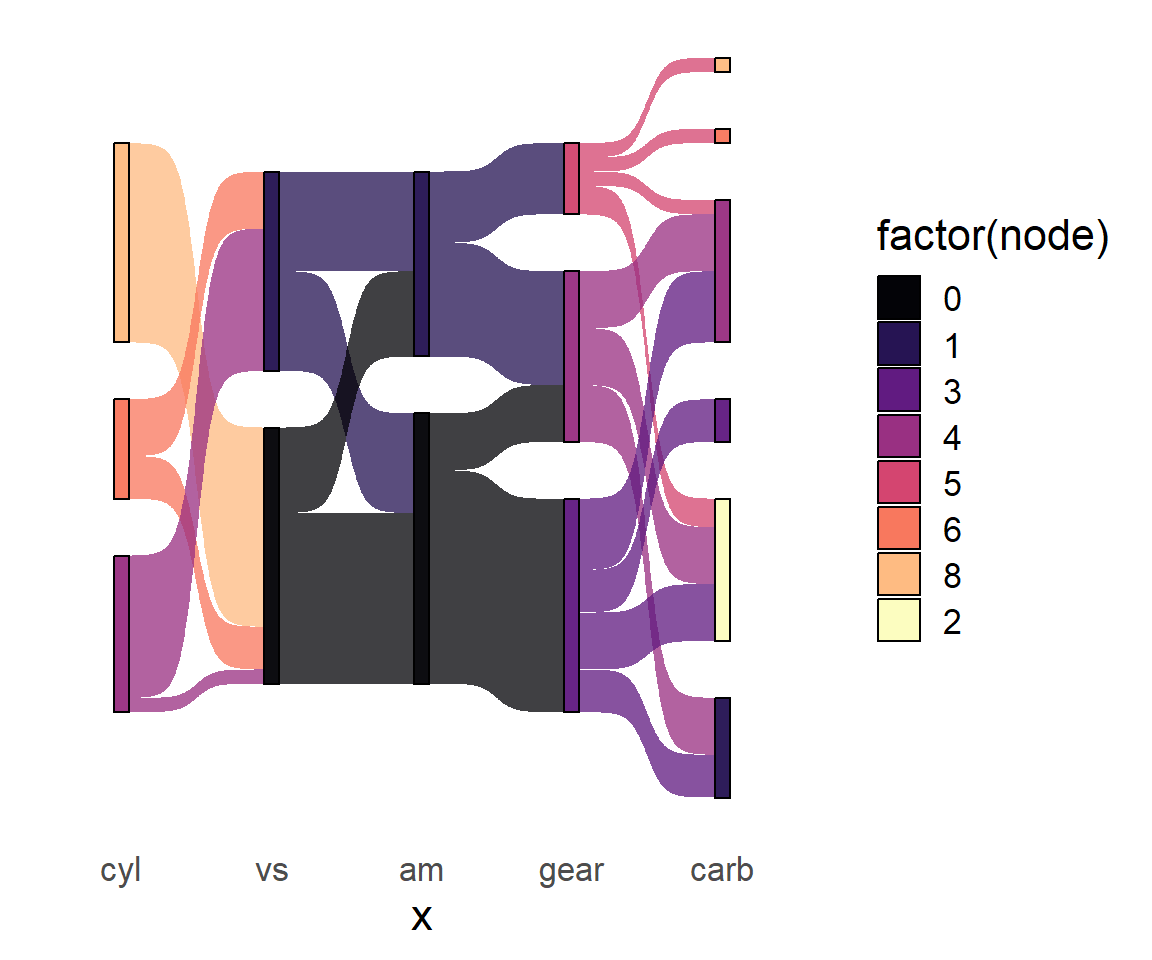``````# install.packages("remotes")
# remotes::install_github("davidsjoberg/ggsankey")
library(ggsankey)
# install.packages("ggplot2")
library(ggplot2)
# install.packages("dplyr")
library(dplyr) # Also needed

ggplot(df, aes(x = x,
next_x = next_x,
node = node,
next_node = next_node,
fill = factor(node))) +
geom_sankey(flow.alpha = 0.75, node.color = 1) +
scale_fill_viridis_d(option = "A", alpha = 0.95) +
theme_sankey(base_size = 16)``````

Changing the color of the labels

Note that the `geom_sankey_label` also contains several arguments to change the appearance of the labels of the plot.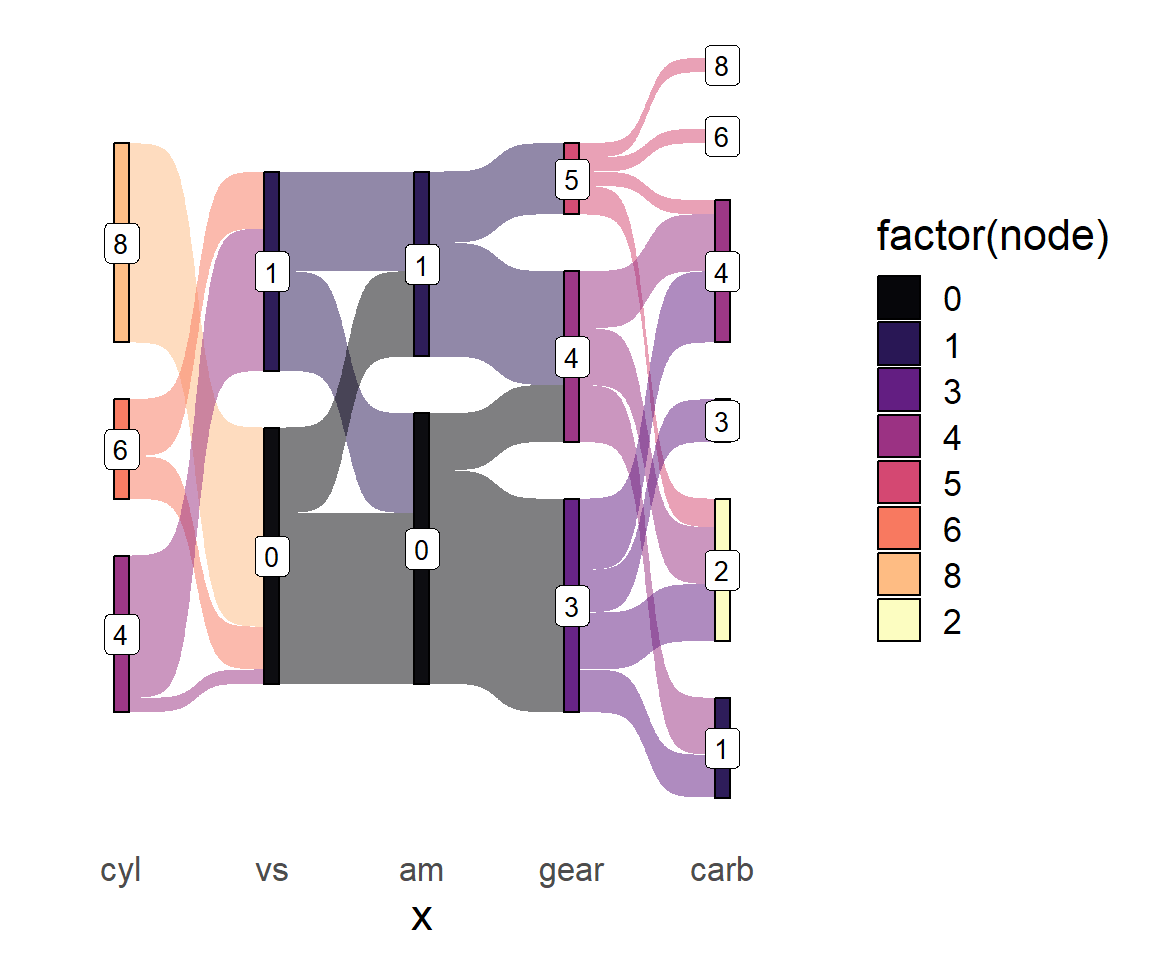``````# install.packages("remotes")
# remotes::install_github("davidsjoberg/ggsankey")
library(ggsankey)
# install.packages("ggplot2")
library(ggplot2)
# install.packages("dplyr")
library(dplyr) # Also needed

ggplot(df, aes(x = x,
next_x = next_x,
node = node,
next_node = next_node,
fill = factor(node),
label = node)) +
geom_sankey(flow.alpha = 0.5, node.color = 1) +
geom_sankey_label(size = 3.5, color = 1, fill = "white") +
scale_fill_viridis_d(option = "A", alpha = 0.95) +
theme_sankey(base_size = 16)``````

## Legend customization

Changing the title of the legend

As with other ggplot2 charts it is possible to change the title of the legend. In this case you can do the following:

``````# install.packages("remotes")
# remotes::install_github("davidsjoberg/ggsankey")
library(ggsankey)
# install.packages("ggplot2")
library(ggplot2)
# install.packages("dplyr")
library(dplyr) # Also needed

ggplot(df, aes(x = x,
next_x = next_x,
node = node,
next_node = next_node,
fill = factor(node),
label = node)) +
geom_sankey(flow.alpha = 0.5, node.color = 1) +
geom_sankey_label(size = 3.5, color = 1, fill = "white") +
scale_fill_viridis_d() +
theme_sankey(base_size = 16) +
guides(fill = guide_legend(title = "Title"))``````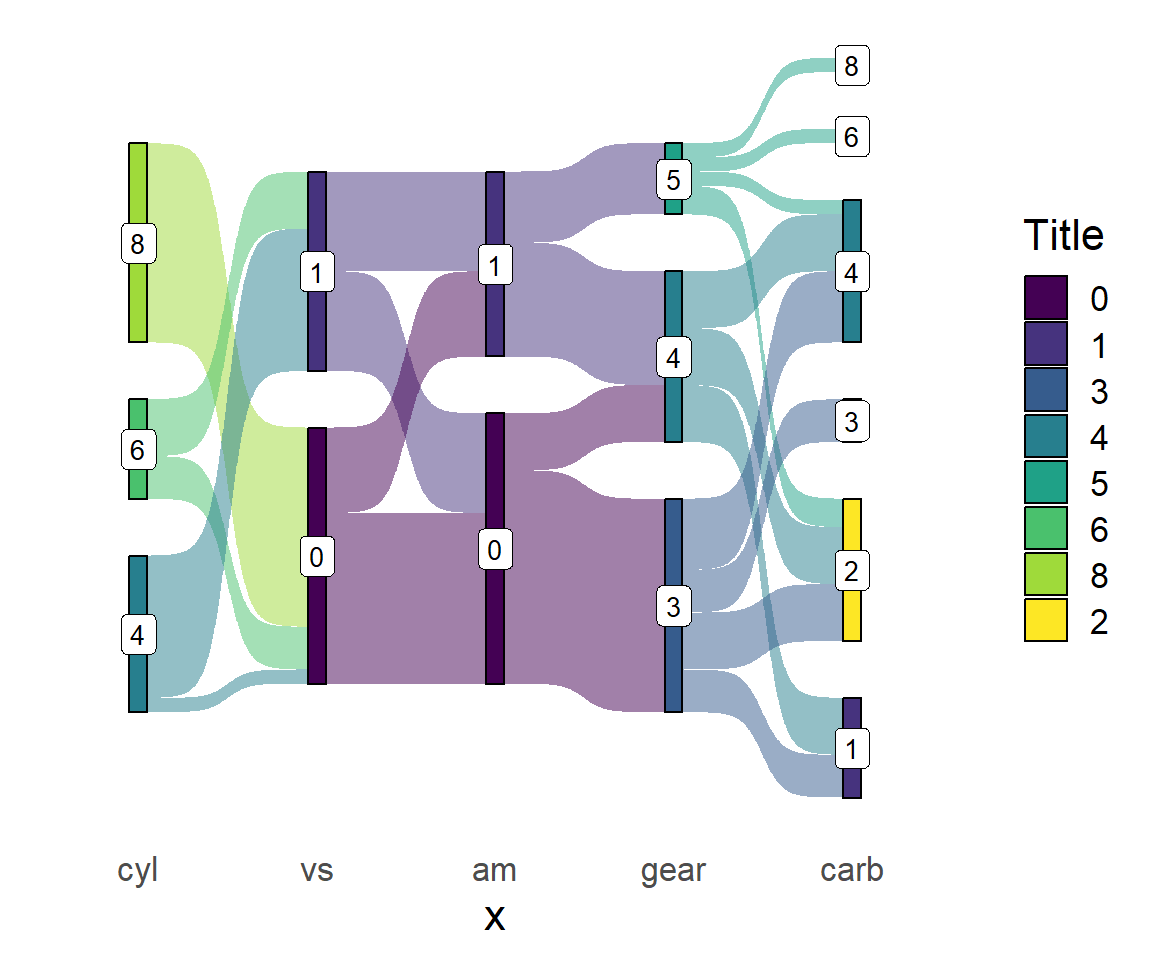Removing the legend

Finally, if you want to get rid of the legend of the Sankey plot you can set its position to `"none"`.

``````# install.packages("remotes")
# remotes::install_github("davidsjoberg/ggsankey")
library(ggsankey)
# install.packages("ggplot2")
library(ggplot2)
# install.packages("dplyr")
library(dplyr) # Also needed

ggplot(df, aes(x = x,
next_x = next_x,
node = node,
next_node = next_node,
fill = factor(node),
label = node)) +
geom_sankey(flow.alpha = 0.5, node.color = 1) +
geom_sankey_label(size = 3.5, color = 1, fill = "white") +
scale_fill_viridis_d() +
theme_sankey(base_size = 16) +
theme(legend.position = "none")``````